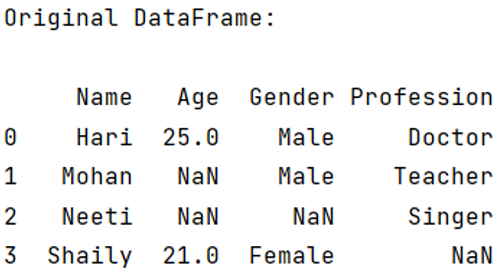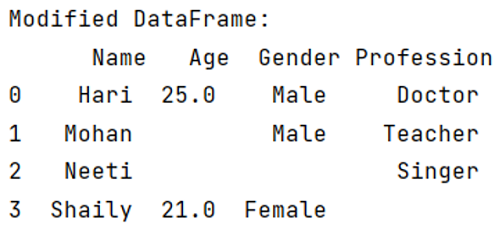# How to replace NaN with blank/empty string?

Learn how to replace NaN with blank/empty string?
Submitted by Pranit Sharma, on May 10, 2022

While creating a DataFrame or importing a CSV file, there could be some NaN values in the cells. NaN values mean "Not a Number" which generally means that there are some missing values in the cell. To deal with this type of data, you can either remove the particular row (if the number of missing values is low) or you can handle these values.

To replace the Nan values with blank strings, we will use dataframe.replace() method.

## pandas.DataFrame.replace() Method

The dataframe.replace() function in Pandas is a simple method that takes two parameters, first is the value of the string, list, dictionary, etc which has to be replaced. Secondly, it takes the value with which our data has to be replaced.

To work with pandas, we need to import pandas package first, below is the syntax:

```import pandas as pd
```

Let us understand with the help of an example:

```# Importing pandas package
import pandas as pd

# To create NaN values, you must import numpy package,
# then you will use numpy.NaN to create NaN values
import numpy as np

# Creating a dictionary with some NaN values
d = {
"Name":['Hari','Mohan','Neeti','Shaily'],
"Age":[25,np.NaN,np.NaN,21],
"Gender":['Male','Male',np.NaN,'Female'],
"Profession":['Doctor','Teacher','Singer',np.NaN]
}

# Now we will create DataFrame
df = pd.DataFrame(d)

# Viewing the original DataFrame
print("Original DataFrame:\n")
print(df,"\n\n")
```

Output:We can clearly see the NaN values in the above output, we need to replace these NaN values with blank string.

```# Replacing NaN values with 0
df = df.replace(np.nan, "")

# Viewing The replaced vales
print("Modified DataFrame:\n",df)
```

Output:Preparation